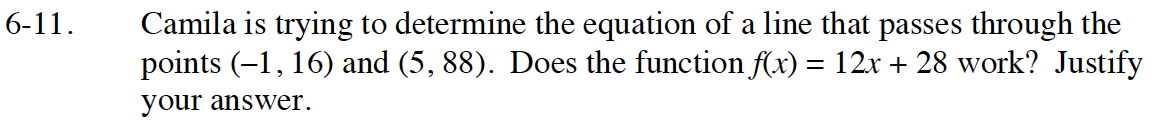Home > GB8I > Chapter cc46 > Lesson cc46.1.1 > Problem6-11

6-11.

Camila is trying to determine the equation of a line that passes through the points (–1, 16) and (5, 88). Does the function f(x) = 12x + 28 work? Justify your answer. Homework Help ✎Substitute both sets of coordinates into the equation.

If both make the equation true, then it is the equation of a line that passes through those two points.